java程序员当前位置：主页 > 高端课程 > java程序员 >

java基础(6):方法

# 1.方法

## 1.2 方法的语法格式

```修饰符 返回值类型 方法名(参数类型 参数名1,参数类型 参数名2,．．．．．．){
执行语句
………
return 返回值;
}```

return关键字：用于结束方法以及返回方法指定类型的值

```public class MethodDemo01 {
public static void main(String[] args) {
int area = getArea(3, 5); // 调用 getArea方法
System.out.println(" The area is " + area);
}

// 下面定义了一个求矩形面积的方法，接收两个参数，其中x为高，y为宽
public static int getArea(int x, int y) {
int temp = x * y; // 使用变量temp记住运算结果
return temp; // 将变量temp的值返回
}
}```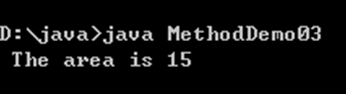## 1.3 方法调用图解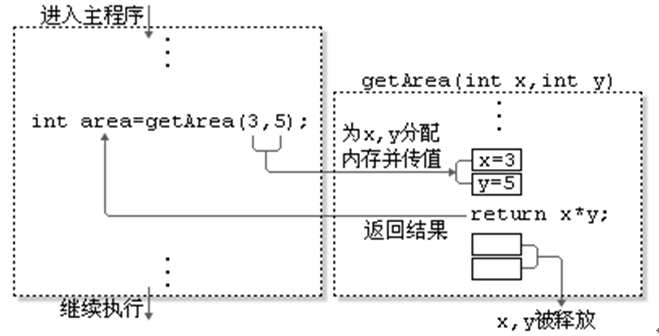## 1.4 方法定义练习

```public static void printRect(){
//打印3行星
for (int i=0; i<3; i++) {
//System.out.println("***"); 相当于是打印3颗星，换行
//每行打印3颗星
for (int j=0; j<3; j++) {
System.out.print("*");  // ***
}
System.out.println();
}
}```

```public static int getNumber(){

Scanner sc = new Scanner(System.in);

int number = sc.nextInt();

return number;

}```

```public static void printRect2(int m, int n){
//打印M行星
for (int i=0; i<m; i++) {
//每行中打印N颗星
for (int j=0; j<n; j++) {
System.out.print("*");
}
System.out.println();
}
}```

```public static double getAvg(double a, double b, double c) {
double result = (a+b+c)/3;
return result;
}```

## 1.5 方法的重载

```public class MethodDemo02 {
public static void main(String[] args) {
// 下面是针对求和方法的调用
int sum2 = add02(1, 2, 3);
// 下面的代码是打印求和的结果
System.out.println("sum1=" + sum1);
System.out.println("sum2=" + sum2);
System.out.println("sum3=" + sum3);
}

// 下面的方法实现了两个整数相加
public static int add01(int x, int y) {
return x + y;
}
// 下面的方法实现了三个整数相加
public static int add02(int x, int y, int z) {
return x + y + z;
}
// 下面的方法实现了两个小数相加
public static double add03(double x, double y) {
return x + y;
}
}```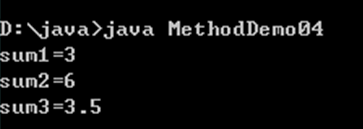```public static int add(int x,int y) {逻辑} //两个整数加法
public static int add(int x,int y,int z) {逻辑} //三个整数加法
public static int add(double x,double y) {逻辑} //两个小数加法```

```public class MethodDemo03 {
public static void main(String[] args) {
// 下面是针对求和方法的调用
int sum2 = add(1, 2, 3);
// 下面的代码是打印求和的结果
System.out.println("sum1=" + sum1);
System.out.println("sum2=" + sum2);
System.out.println("sum3=" + sum3);
}

// 下面的方法实现了两个整数相加
public static int add(int x, int y) {
return x + y;
}
// 下面的方法实现了三个整数相加
public static int add(int x, int y, int z) {
return x + y + z;
}
// 下面的方法实现了两个小数相加
public static double add(double x, double y) {
return x + y;
}
}```

MethodDemo02.java的运行结果和MethodDemo03.java一样，如下图所示。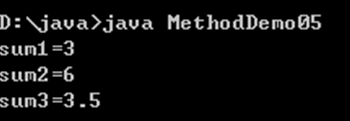### 1.5.1     重载的注意事项

参数个数不同，如method(int x)与method(int x,int y)不同

参数类型不同，如method(int x)与method(double x)不同g

参数顺序不同，如method(int x,double y)与method(double x,int y)不同

l重载只与方法名与参数类型相关与返回值无关：

如void method(int x)与int method(int y)不是方法重载，不能同时存在

如method(int x)与method(int y)不是方法重载，不能同时存在

### 1.5.2 参数传递

```public class ArgumentsDemo01 {
public static void main(String[] args) {
int a=5;
int b=10;
change(a, b);//调用方法时，传入的数值称为实际参数
System.out.println("a=" + a);
System.out.println("b=" + b);
}

public static void change(int a, int b){//方法中指定的多个参数称为形式参数
a=200;
b=500;
}
}```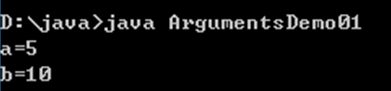```public class ArgumentsDemo02 {
public static void main(String[] args) {
int[] arr = { 1, 2, 3 };
change(arr);// 调用方法时，传入的数值称为实际参数

for (int i = 0; i < arr.length; i++) {
System.out.println(arr[i]);
}
}

public static void change(int[] arr) {// 方法中指定的多个参数称为形式参数
for (int i = 0; i < arr.length; i++) {
arr[i] *= 2;
}
}
}    ```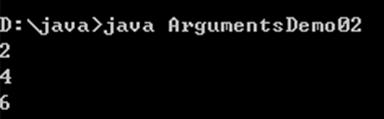### 1.5.3 参数传递图解与结论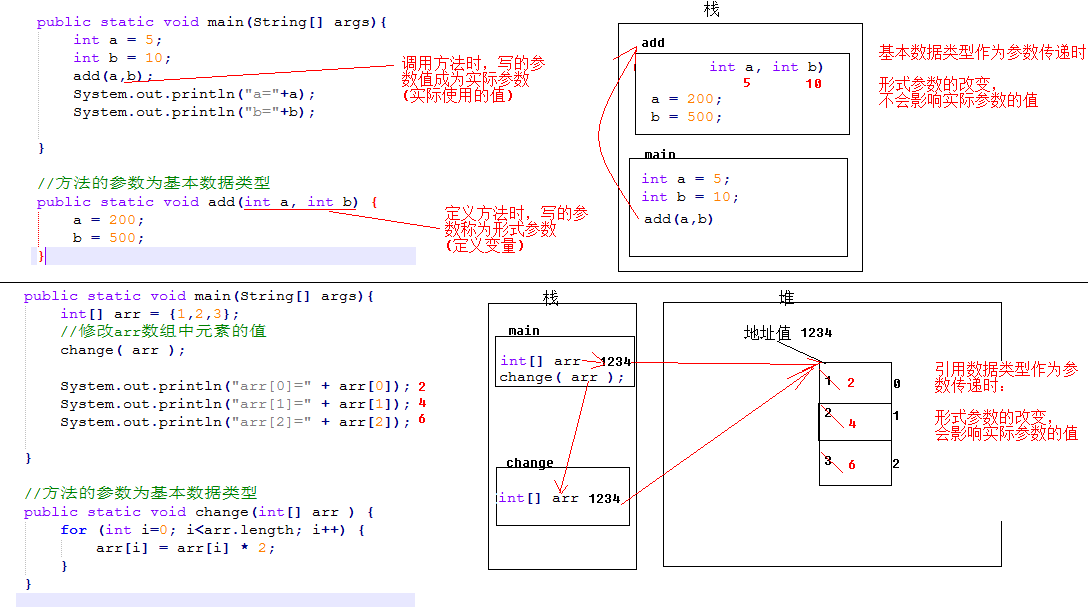• 全球最大晶圆代工半导体制造厂，台积电斥资订购艾斯摩尔机器设备
• 英特尔依然是那个英特尔，且看英特尔的城防体系
• 支持双 DRAM 内存接口，慧荣企业级 SSD 主控方案披露
• 在全球被反垄断罚款，冤！高通到底哪里得罪了欧盟？
• 强强联合！万业、微电子所和芯鑫共同打造全新半导体设备
• 复旦大学校长称：对于集成电路产业发展，大学应该主动担当
• 技术再升级！无锡中科芯攻克晶圆级再布线及晶圆级凸点制备关键技
• 聚力！万业企业设立集成电路装备集团，提供自主可控设备
• 德州仪器C2000微控制器增强连通性和控制性
• 英特尔打出降价策略，以免被竞争对手 AMD 打败？
• 贸易摩擦的闹剧没有赢家，苹果有勇气离开中国吗？
• 图像信号与视觉处理器的发展趋势
• 真干快消品定位方案班（第二期）火热开班
• Java集合 ArrayList原理及使用
• TDD（测试驱动开发）死了吗？
• JAVA基础之XML相关
• javaweb项目搭建ehcache缓存系统
• 每日一码——字符串统计
• 一篇文章帮你彻底搞清楚“I/O多路复用”和“异步I/O”的前世今生
• 九：模板方法模式
• 十二：命令模式（人员解耦和）
• Java 转PPT为图片、PDF、SVG、XPS、ODP以及PPT和PPTX互转
• SpringCloud学习（SPRINGCLOUD微服务实战）一
• 记一次微信网页授权后获取用户信息并重定向
• 速途新营销五点实战洞察解码“品效合一”
• 十一：外观模式详解（Service，action与dao）
• 手把手教你学会 基于JWT的单点登录
• mysql锁机制总结，以及优化建议
• 解决多个版本jar包冲突【jar内包名重命名】
• 中国首张5G终端电信设备进网许可证 华为Mate 20 X 5G版入网
• RPC之Thrift
• 高级Java工程师必备 ----
• 天猫618期间实物支付GMV增长38.5%
• 换季了，老板你的库存处理好了吗？
• 从“618”大数据看中国消费新活力
• 小米生态链：贵在格局感与收放度
• CODING 2.0 企业级持续交付解决方案
• 老铁奇趴“新京济” 快手*京东618战报出炉
• 中小企业新媒体运营基本技能
• 上汽大通房车再度携手LINE FRIENDS 魔都巡游顺利开启
• 华为高端手机国内市场份额超苹果夺得榜首
• 中国智能制造分析报告
• iPlus艾加营销助力腾讯广告牵手吴晓波 推进商业IP变现
• 2019世界新能源汽车大会7月1日将在海南举行
• 区域酒企如何转型突围
• 时时彩论坛
• 五星体育斯诺克
• 北单比分直播
• 河北11选5走势图
• 福建体彩36选7开奖结果
• 九龙图库下载